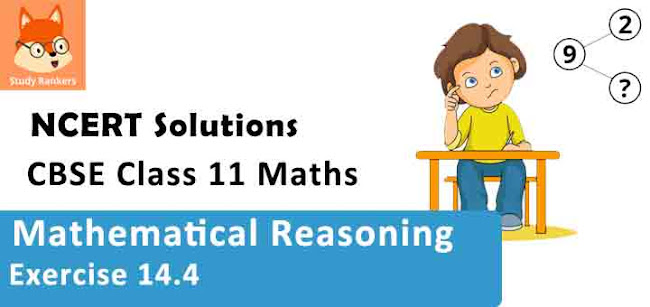# Class 11 Maths NCERT Solutions for Chapter 14 Mathematical Reasoning Exercise 14.4### Mathematical Reasoning Exercise 14.4 Solutions

1. Rewrite the following statement with “if-then” in five different ways conveying the same meaning.
If a natural number is odd, then its square is also odd.

Solution

The given statement can be written in five different ways as follows.
(i) A natural number is odd implies that its square is odd.
(ii) A natural number is odd only if its square is odd.
(iii) For a natural number to be odd, it is necessary that its square is odd.
(iv) For the square of a natural number to be odd, it is sufficient that the number is odd.
(v) If the square of a natural number is not odd, then the natural number is not odd.

2. Write the contrapositive and converse of the following statements.
(i) If x is a prime number, then x is odd.
(ii) It the two lines are parallel, then they do not intersect in the same plane.
(iii) Something is cold implies that it has low temperature.
(iv) You cannot comprehend geometry if you do not know how to reason deductively.
(v) x is an even number implies that x is divisible by 4

Solution

(i) The contrapositive is as follows.
If a number x is not odd, then x is not a prime number.
The converse is as follows.
If a number x is odd, then it is a prime number.

(ii) The contrapositive is as follows.
If two lines intersect in the same plane, then they are not parallel.
The converse is as follows.
If two lines do not intersect in the same plane, then they are parallel.

(iii) The contrapositive is as follows.
If something does not have low temperature, then it is not cold.
The converse is as follows.
If something is at low temperature, then it is cold.

(iv) The contrapositive is as follows.
If you know how to reason deductively, then you can comprehend geometry.
The converse is as follows.
If you do not know how to reason deductively, then you cannot comprehend geometry.

(v) The given statement can be written as follows.
If x is an even number, then x is divisible by 4.
The contrapositive is as follows.
If x is not divisible by 4, then x is not an even number.
The converse is as follows.
If x is divisible by 4, then x is an even number.

3. Write each of the following statement in the form “if-then”.
(i) You get a job implies that your credentials are good.
(ii) The Banana trees will bloom if it stays warm for a month.
(iii) A quadrilateral is a parallelogram if its diagonals bisect each other.
(iv) To get A+ in the class, it is necessary that you do the exercises of the book.

Solution

(i) If you get a job, then your credentials are good.
(ii) If the Banana tree stays warm for a month, then it will bloom.
(iii) If the diagonals of a quadrilateral bisect each other, then it is a parallelogram.
(iv) If you want to get an A+ in the class, then you do all the exercises of the book.

4. Given statements in (a) and (b). Identify the statements given below as contrapositive or converse of each other.
(a) If you live in Delhi, then you have winter clothes.
(i) If you do not have winter clothes, then you do not live in Delhi.
(ii) If you have winter clothes, then you live in Delhi.
(b) If a quadrilateral is a parallelogram, then its diagonals bisect each other.
(i) If the diagonals of a quadrilateral do not bisect each other, then the quadrilateral is not a parallelogram.
(ii) If the diagonals of a quadrilateral bisect each other, then it is a parallelogram.

Solution

(a) (i) This is the contrapositive of the given statement (a).
(ii) This is the converse of the given statement (a).

(b) (i) This is the contrapositive of the given statement (b).
(ii) This is the converse of the given statement (b).